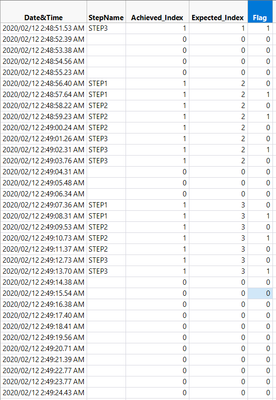The World Statistics Day celebration continues here in the Community. We all need reliable data for sound decision making. Do you have a data source that you trust most? Head over to Discussions to tell us about it.
Choose Language Hide Translation Bar
Highlighted

## Index that enumerates by group

Hello,

I'm trying to setup an index that enumerates by a group or set of rows. I've attached a picture to try to explain better what I'm trying to achieve.

As you can see on the picture I'm trying to get the index to be based on the group of steps. The difficulty here or what I'm finding difficult is that the number of instances each step is mentioned for each group can vary and there are gaps where none are listed.This is what I've used to get me as far as getting the results shown in :Achieved_Index but what I'm really looking for is results like in :Expected_Index

``````dt = Data Table("sample");
dt << New Column( "Achieved_Index", Numeric( ) );

x = 1;
For Each Row( If( :StepName == "",
:Achieved_Index = 0,
If( Lag(:StepName, -1) != "" | :StepName == "STEP3",
:Achieved_Index = x,
x = x + 1
)
)
);``````

The ultimate goal is to get that index column working correctly. Than using it to setup the flag column to identify the half-way mark or the last step for each group if there are only two instance. I haven't really thought much on this one besides possibly getting the count of each step for each index, dividing by 2 than using this value for my Lag( ) function. Any suggestions on this would also be appreciated thank you!

1 ACCEPTED SOLUTION

Accepted Solutions
Highlighted

## Re: Index that enumerates by group

txnelson, thanks for the help. This helped me get to where I needed.

This is the code that performed what I was looking for:

``````For Each Row(
If( Row() == 1, step = 0);
If(Row() == 1 & :StepName != "", step = 1);
If( :StepName != "" & Lag( :StepName ) == "",
:Achieved_index = step++);
If( :StepName != "", :Achieved_index = step, :Achieved_index = 0 );
);``````

I had to make a couple of edits/additions because even though I'm calling it an index I wanted to start at 1.

I also had to add an additional If statement because if the first row had :StepName == "" it would throw off the count.

2 REPLIES 2
Highlighted

## Re: Index that enumerates by group

The formula below will get you the answers you want

``````If( Row() == 1, step = 0 );
If( :stepName != "" & Lag( :stepName ) == "" | Row() == 1,
step
++);
If( :stepName != "", step, 0 );``````
Jim
Highlighted

## Re: Index that enumerates by group

txnelson, thanks for the help. This helped me get to where I needed.

This is the code that performed what I was looking for:

``````For Each Row(
If( Row() == 1, step = 0);
If(Row() == 1 & :StepName != "", step = 1);
If( :StepName != "" & Lag( :StepName ) == "",
:Achieved_index = step++);
If( :StepName != "", :Achieved_index = step, :Achieved_index = 0 );
);``````

I had to make a couple of edits/additions because even though I'm calling it an index I wanted to start at 1.

I also had to add an additional If statement because if the first row had :StepName == "" it would throw off the count.

Article Labels

There are no labels assigned to this post.